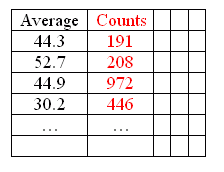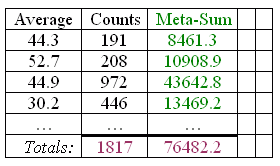Name: Ray Who is asking: Other Level of the question: All Question: I have the averages of some data in percentages. the data is rather large, how can i find the average of these averages without going back to the original data? Is there a Factor or Constant that i could multiply or devide or add and get the average of these averages without resorting to the raw data. Hi Ray. If you have also recorded the number of data points represented by each average (just the total count), then you can multiply each average by its corresponding data point count. For example, here is a table of averages with a red column added recording the data counts represented in each average:If you have this information, you can add a third column (which I denote "Meta-Sum") which is the average times its data count. Then you can get totals for these two columns:If you then divide the total Meta-Sum by the total Count, you get an average for all your original data. Hope this helps! Stephen La Rocque> Just to make sure that we understood Ray's question I asked for more information and he sent: Consider this scenario; I have the percentage number hours an equipment worked in a day for one month, and I obtain the average number of hours worked in a month in percentage. Then I have the same figures for January, February, March and April which all have different number of days. All I have is the average number of hours worked in percentage and I want to find the average for the first quarter, using only the monthly percentages without having to resort to all the hours run each day. Ray, Your situation does fit Sue's answer. The "Average" column is your average of the percentage of the day the equipment worked in a month. The "Counts" column is the number of days in the month (or the number of working days, whatever you divided by to find the average in the first column). The sum of the "Meta-Sum" column is the the number of days (or working days) in the first quarter. Harley